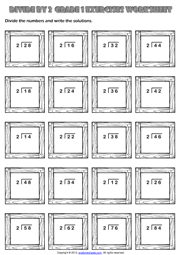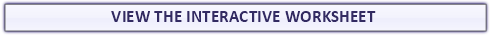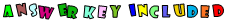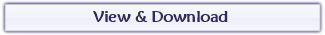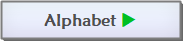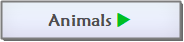Found a mistake?
!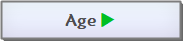## First Grade Easy Division Exercise Maths Worksheet

A fun and easy division exercise maths worksheet for grade 1 (first grade) students and kids with trainers theme. Divide the numbers and write the solutions. (Horizontal Division Worksheet for kids single and double digits)## Divide by 2 Maths Exercise Worksheet With Two Digits

An easy division exercise maths worksheet without remainders for grade 1 (first grade) students and kids with wooden frames theme. Divide the numbers by two and write the solutions. (Long Division Worksheet for kids)